# Lecture #2: Directed Graphs - Transition Matrices

A graph is an object that consists of a non-empty set of vertices and another set of edges. When working with real-world examples of graphs, we sometimes refer to them as networks. The vertices are often called nodes or points, while edges are referred to as links or lines. The set of edges may be empty, in which case the graph is just a collection of points.

Example 1: A simple example of a graph with vertices 1,2,3,4, and directed edges from vertex 1 to vertex 2, vertex 3 to vertices 2 and 4, and vertex 4 to vertex 1.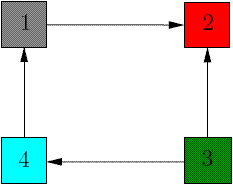In this lecture we will only work with directed graphs and real-world examples of those (Internet graphs), but for other properties of graphs we refer to Math Explorer's Club website. The central example in this module is the web graph, in which web pages are represented as vertices and the links between them are represented as edges. An example of such a graph is a sub graph of the BGP (Gateway Protocol) web graph, consisting of major Internet routers. It has about 6400 vertices and 13000 edges and it was produced by Ross Richardson and rendered by Fan Chung Graham.

Although Internet graphs are very large, having the number of vertices of the order 30 billion (and growing), all graphs in this module are considered finite (finite number of vertices and edges).

We say that two vertices i and j of a directed graph are joined or adjacent if there is an edge from i to j or from j and i. If such an edge exists, then i and j are its endpoints. If there is an edge from i to j then i is often called tail, while j is called head. In Example 1, vertices 1 and 2 are joined because there is an edge from 1 to 2, while vertices 1 and 3 are not joined. There is however no edge from node 2 to node 1. Notice that there can be no more than two edges between any two vertices. There is a strong relation between graphs and matrices, previously introduced in Lecture 1. Suppose we are given a directed graph with n vertices. Then we construct an n × n adjacency matrix A associated to it as follows: if there is an edge from node i to node j, then we put 1 as the entry on row i, column j of the matrix A.

Example 2: Adjacency matrix for the graph in Example 1: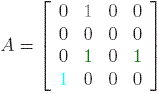.

If one can walk from node i to node j along the edges of the graph then we say that there is a path from i to j. If we walked on k edges, then the path has length k. For matrices, we denote by Ak the matrix obtained by multiplying A with itself k times. The entry on row i, column j of A2 = A·A corresponds to the number of paths of length 2 from node i to node j in the graph. For Example 2, the square of the adjacency matrix is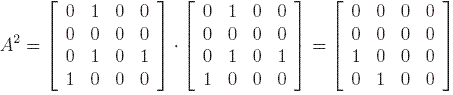This means that there is a path from vertex 4 to vertex 2, because the entry on fourth row and second column is 1. Similarly there is a path from 3 to 1, as one can easily see from Example 1.

Fact 1: Consider a directed graph and a positive integer k. Then the number of directed walks from node i to node j of length k is the entry on row i and column j of the matrix Ak, where A is the adjacency matrix.

In general, a matrix is called primitive if there is a positive integer k such that Ak is a positive matrix. A graph is called connected if for any two different nodes i and j there is a directed path either from i to j or from j to i. On the other hand, a graph is called strongly connected if starting at any node i we can reach any other different node j by walking on its edges. In terms of matrices, this means that if there is a positive integer k such that the matrix B = I + A + A2 + A3 + … +Ak is positive, then the graph is strongly connected. We add the identity matrix I in order to deal with edges from a vertex to itself. In other words, if there is at least one path from node i to node j of length at most k, then we can travel from node i to j. Thus if matrix B has a positive entry on row i and column j then it is possible to reach node j starting from i. If this happens for all nodes, then the graph is strongly connected.

One can easily see that the graph in Example 1 is connected, but not strongly connected because there is no edge from vertex 1 to vertex 3. For the matrix in Example 2, we notice that A4 is a matrix having only zeros, and so for all k greater than 4, Ak will be a matrix filled with zeros. Then for any k greater than 4, the matrix B = I + A + A2 + A3 + … +Ak is :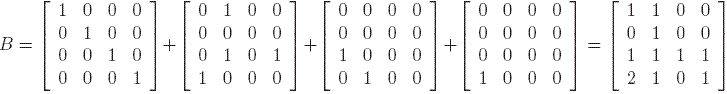Since the matrix B is not positive, the graph in Example 1 is not strongly connected as we already saw.

Problem 1: Construct the adjacency matrix of the graph below. Is this graph connected or strongly connected? How many paths of length 3 from node 3 to node 2 are there?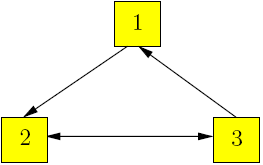In the examples above we noticed that for every vertex i there is a number of edges that enter that vertex (i is a head) and a number of edges that exit that vertex (i is a tail). Thus we define the indegree of i as the number of edges for which i is a head. Similarly, the outdegree of i as the number of edges for which i is a tail. For example, for the graph in the Problem 1, the indegree of node 2 is 2 and the outdegree of node 1 is 1. The transition matrix A associated to a directed graph is defined as follows. If there is an edge from i to j and the outdegree of vertex i is di, then on column i and row j we put. Otherwise we mark column i, row j with zero. Notice that we first look at the column, then at the row. We usually writeon the edge going from vertex i to an adjacent vertex j, thus obtaining a weighted graph. This will become clear through the following example.

Example 3: Consider the graph from Example 1 with weights on its edges as described above.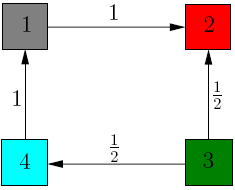Then the transition matrix associated to it is:Notice that the sum of the entries on the first column is 1. The same holds for the third and fourth column. In general, more is true.

Fact 2: For a strongly connected graph, the transition matrix is column-stochastic.

We use the transition matrix to model the behavior of a random surfer on a web graph. The surfer chooses a page at random, then follows its links to other web pages for as long as he/she wishes. At each step the probability that the surfer moves from node i to node j is zero if there is no link from i to j andotherwise. Recall that di is the outdegree of vertex i. Initially the probability of each page to be chosen as a starting point is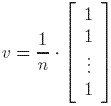At step 1, the probability of each node to be visited after one click is A·v. At step 2, the probability of each node to be visited after two clicks is A2·v. The probability of a page to be visited at step k is thus Ak·v. If the matrix is primitive, column-stochastic, then this process converges to a unique stationary probability distribution vector p, whereThe meaning of the ith entry of p is that the surfer visits page i at any given time with probability pi.

Problem 2: Let A be a transition matrix associated with a graph and B be a matrix of size n filled with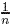. Consider x a positive real number smaller than 1. Then the matrix C = x·A + (1-x)·B is column-stochastic. Show that this is true in the special case of Problem 1.